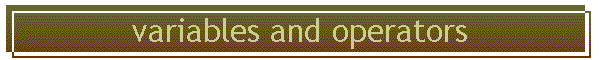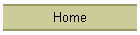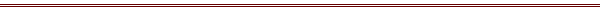These are mostly similar to those in Fortran.  Arrays and structures are fundamental data types.  The second is not found in F77.

## variable names

C is case sensitive!  This is why Unix is case sensitive.  The variables chChar and chchar are not the same.

## variable types

All functions are double precision in C.  For most processors this brings no speed penalty.  It will probably be slower to have single precision variables and have to recast the double precision function values to single precision.

 Fortran C integer integer long single precision float read float double precision float double double character strings character char (arrays only, no string type)

There are two other integer types, although these are of lesser value in large-scale scientific calculations.  Integers can also be short, and unsigned.  There are no complex variables, although I have never had to use complex numbers in 28 years of coding Fortran.

## scope of variables

There are two main classes, local and global namespace.  In the following example the variable "global" can be seen by any other routine that declares it, while the variable "local" is only available to code inside the brackets:

/* this variable is global */
double global = 0.;
double MyRoutine( void )
{
/* local variable */
long local = 0.;
code goes here  . .. .;
return ;
}

## static (C) and save (Fortran)

A local variable is redefined from the stack when this part of a code is entered.   Its value will be undefined, unless it is saved by declaring the variable to be "static".  The equivalent statement in Fortran is "save".  N.B., few Fortran compiler actually use stack memory, and save statements are not important.  All the C compilers I have seen take this very seriously - variable values will be volatile and lost if not declared static.

In the following code fragment:
double a=0.;
void routine( double b )
{
static double c=0;
double d = 0;
double e;
c = a + d;
}
a is a global variable and never looses its value.  c is a local static variable, and is equal to 0 the first time the routine is called, and retains whatever value it is given.  d is a volatile local variable, and is reset to 0 every time the function is entered.  e is a volatile local variable whose value is unspecified when the routine is called.

## common blocks, block data, definition, declaration

In Fortran a variable is made available to other routines by placing it into common.   This variable can be initialized with either a block data routine, or by setting it equal to a value.  Commons and block datas do not exist in C.

defining a variable

A variable must be defined in exactly one place in a code to allocate memory for it. The variable can be initialized at the same time it is defined.  The following defines and initializes the variable Temperature:

double Temperature = 0;

declaring a variable

If this is in the global namespace (appears before the first open bracket in the routine where it occurs) then other routines can access the variable by declaring it with the extern keyword:

extern double Temperature;

A deFinition Fixes the variable in memory.  A declaration is like a customs declaration - you say what is in that bag over there.

## array syntax and limits

An array is written array[i].  A multidimensional array is array[i][j], which looks funny from a Fortran context.  There is no limit to the number of dimensions an array can have.

A multidimensional array is really an array of arrays.

Arrays start from 0.  It is dimensioned when it is defined:
double array;
will go from array to array.  This is why "for" loops in C nearly always go from 0 to less than a limit.  The following uses the preprocessor to set the token LENGTH to a value, then declare a vector, and set it to zero in a loop:

#define LENGTH 10
double array[LENGTH];
long i; /*this will be the loop index */
/* now set it to zero */
for( i=0; i<LENGTH; i++ )
{
array[i] = 0.;
}

## initializing an array

Any variable can have its value set when the variable is defined.  For an array with one dimension it would be initialized with a single set of brackets:
long nRow = 10;
double array[nRow] = {1.,2.,1.,2.,1.,2.,1.,2.,3.,4.};

Two dimensional arrays are done by analogy.
long nRow = 10 , nCol=2;
double array[nRow][nRow] = {
{1.,2.,1.,2.,1.,2.,1.,2.,3.,4.} ,
{1.,2.,1.,2.,1.,2.,1.,2.,3.,4.}    }

If you do not give an explicit dimension, but set the array to a value, the compiler will set the limit for you.  This is only useful for character strings, which you know will be terminated with a null character:
char chString[] = "this is a string";

## Row vs column major

This determines how arrays are laid out in memory.   Fortran is column major, so that the leftmost subscript varies the fastest in memory.  Most other languages, C included, are row major so the rightmost index varies fastest.

Two ways to remember this - an two dimensional array is like a Roman Catholic, Row then Column, and it varies the same way that a car's odometer does.

The following show how two arrays vary from lowest to highest address, in C and Fortran.

 C double a a a a a a a Fortran double a(2.3) a(1.1) a(2,1) a(1.2) a(2,2) a(1,3) a(2,3) location 0 1 2 3 4 5

## malloc and a 1 dimensional array

Here is how to define a simple 1-D array.  First define a pointer, then allocate space as an array.

#include <stdlib.h>
#include <stdio.h>
#include <math.h>
#define NCELL 9000 /* want array with 9000 cells */
/* following will be the array itself */
double *xLines;

void main ( int argc , char *argv[] )
{
void testit1( void);
long n,i;

/* make the space */
xLines = ((double *)malloc(NCELL*sizeof(double )));
if( xLines == NULL )
{
fprintf(stdout,"malloc error \n" );
exit;
}
}

Other routines would access this array as follows

#include <stdlib.h>
#include <stdio.h>
#include <math.h>
extern double *xLines;
void testit2( void )
{
printf("%3i %3i %f \n",4,1 , xLines );
return;
}

## malloc and a 2 dimensional array

Here is how to define a 2-D array - define a pointer to a pointer, then set up an array of pointers, then add a column to each row.

#include <stdlib.h>
#include <stdio.h>
#include <math.h>
/* these are the numbers of rowas and columns in the array */
#define NROW 9
#define NCOL 2
/* this will become xLines[NROW][NCOL] - there are as many
* stars in the follows, as there are dimensions in the final array */
double **xLines;

void main ( int argc , char *argv[] )
{
void testit3( void);
long n,i;

/* first declare row of double pointers -  you always make the
* space from left to right across the array */
xLines = ((double **)malloc(NROW*sizeof(double *)));
if( xLines == NULL )
{
fprintf(stdout,"malloc error \n" );
exit;
}

for (n=0; n < NROW; n++)
{
xLines[n] = (double *)malloc(NCOL*sizeof(double ));
if( xLines[n]  == NULL )
{
fprintf(stdout,"malloc error \n" );
exit;
}
}

Other routines would then refer to this array as follows:

#include <stdlib.h>
#include <stdio.h>
#include <math.h>
extern double **xLines;
void testit4( void )
{
printf("%3i %3i %f \n",4,1 , xLines );
return;
}

This example also shows why array bounds checking is not part of C - the compiler cannot possibly determine the size of xLines  from the second piece of code.   You need to explicitly write code to check the range.

## each malloc should have a free

Memory is allocated from the machine's heap.  If a routine obtains more memory with malloc each time it is entered, then the amount of memory used will gow without bounds.  This is called a memory leak.  (One improvement in Java over C/C++ is that when a routine exists and the memory is no longer needed, the system automatically deallocates it - this is called garbage collection).  In C you must free up the memory allocated with malloc when it is no longer needed.  This is done by calling routine free() with the single argument the name of the variable with the memory to be freed.  In the 2-D example above, you would do this with

for (n=0; n < NROW; n++)
{
free(xLines[n]);
}
free(xLines);

## structures

Structures are one of the most powerful data types that come in with C.  Fortran 90 does support structures, but Fortran 77 does not.

Two dimensional arrays allow you to have both a row and column, but all are of the same data type.  Structures allow you to declare many different types.

An emission line might have an intensity, wavelength, and a pointer to its location within the continuum array.

### defining a structure variable

A structure for the CIV 1549 line might be declared like this:
struct {
double xIntensity , /* the line's intensity */
double Wavelength , /* the wavelength of the line */
long ipContinuum /* the pointer within the continuum array */
} CIV1459 ;

The three variables within the brackets are fields.  The last term is the variable.  Then the wavelength would be CIV1549.Wavelength, or variable.field.

### defining a structure name

The structure above did not have a name, but the variable did.  This structure can only be used with the one explicit definition.  Structures can be given a name, a parameter that appears before the first bracket.  Here is the same structure as an example that does not define a variable, but does define a structure named EmLines:
struct EmLines {
double xIntensity , /* the line's intensity */
double Wavelength , /* the wavelength of the line */
long ipContinuum /* the pointer within the continuum array */
}  ;

This defines a class of structures but there is no variable that has these properties.   With this definition the variable CIV1549 would defined as
struct EmLines CIV1549;

### an array of structures

You can define an array of structures, such as
struc EmLines xLines;
which would define an array xLines, each member being a structure of type EmLines.   One would then reference a wavelength as, fur example,
xLines.Wavelength;

### typedef and struc

These two are very often used together.  typedef defines a new type of variable.   For instance, the following typedef
typedef long LOGICAL;
would allow you to define new variables as
LOGICAL lgOK;

A very common use is
typedef struct EmLines {
double xIntensity , /* the line's intensity */
double Wavelength , /* the wavelength of the line */
long ipContinuum /* the pointer within the continuum array */
}
so that later definitions could say simply
EmLines CIV1549;

I do not use typedef since this hides the basic definition of the data type.  When you see the results, you then must go find the typedef to see what is happening.

## malloc and a structure

#include <stdio.h>
#include <stdlib.h>

/* the definition of the variables of a structure, but no space allocated for it */
struct EmLine {
float a;
int ip;
} ;

/* this says that Level2 is a pointer to variables of this structure */
struct EmLine *Level2 ;

int main(void)
{
/* this will make Level2 and array with 100 structs */
Level2 = malloc( 100*sizeof( struct EmLine ) );
if( Level2 == NULL )
{
printf(" alloc error\n" );
}
Level2.a = 0.;
Level2.ip = 0;
}

## Unions and enum

Fortran has no counterpart to these two data types, and i can think of no need for them in a large-scale code like Cloudy.  See a good book on C if you need to find out what these are.

## Array bounds checking in C++

The lack of standard array bounds checking is the biggest flaw in ANSI C for numerical work.  Here is a description of how to incorporate bounds checking in C++, written by Frank Soloman of UK's McVey Hall.

The subscript operator ([ ]), like the function-call operator, is considered a binary operator. The subscript operator must be a nonstatic member function that takes a single argument. This argument can be of any type and designates the desired array subscript.

The following example demonstrates how to create a vector of type int that implements bounds checking:

#include <iostream.h>

class IntVector
{
public:
IntVector( int cElements );
~IntVector() { delete _iElements; }
int& operator[]( int nSubscript );
private:
int *_iElements;
int _iUpperBound;
};

// Construct an IntVector.
IntVector::IntVector( int cElements )
{
_iElements = new int[cElements];
_iUpperBound = cElements;
}

// Subscript operator for IntVector.
int& IntVector::operator[]( int nSubscript )
{
static int iErr = -1;

if( nSubscript >= 0 && nSubscript < _iUpperBound )
return _iElements[nSubscript];
else
{
clog << "Array bounds violation." << endl;
return iErr;
}
}

// Test the IntVector class.
int main()
{
IntVector v( 10 );

for( int i = 0; i <= 10; ++i )
v[i] = i;

v = v;

for( i = 0; i <= 10; ++i )
cout << "Element: [" << i << "] = " << v[i] << endl;

return v;
}

When i reaches 10 in the preceding program, operator[] detects that an out-of-bounds subscript is being used and issues an error message.

Note that the function operator[] returns a reference type. This causes it to be an l-value, allowing you to use subscripted expressions on either side of assignment operators.